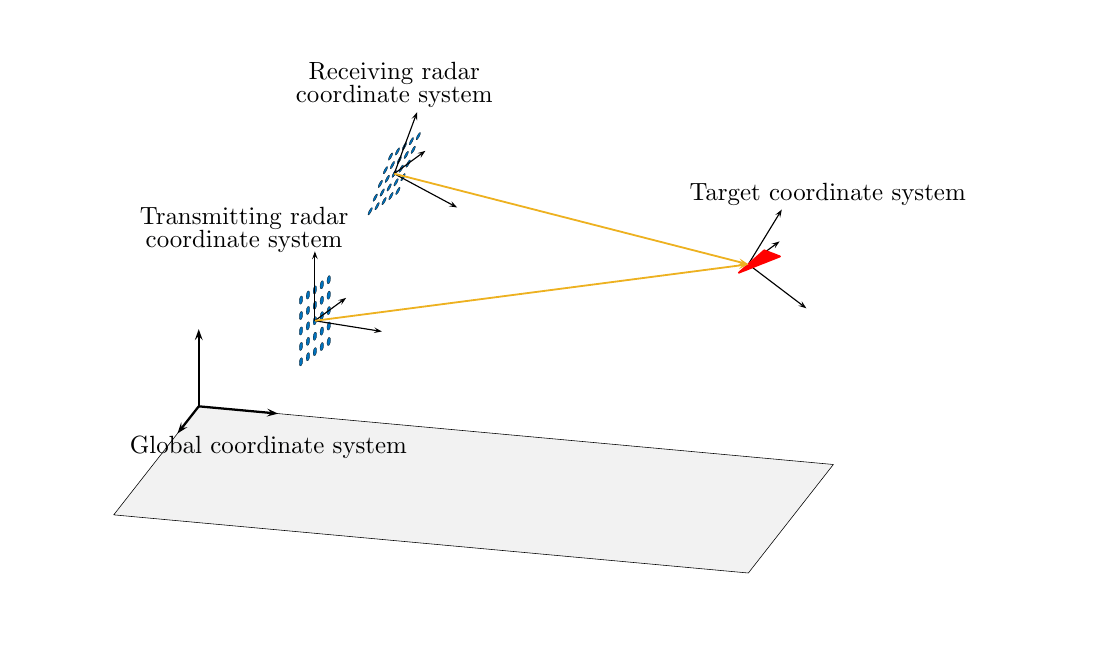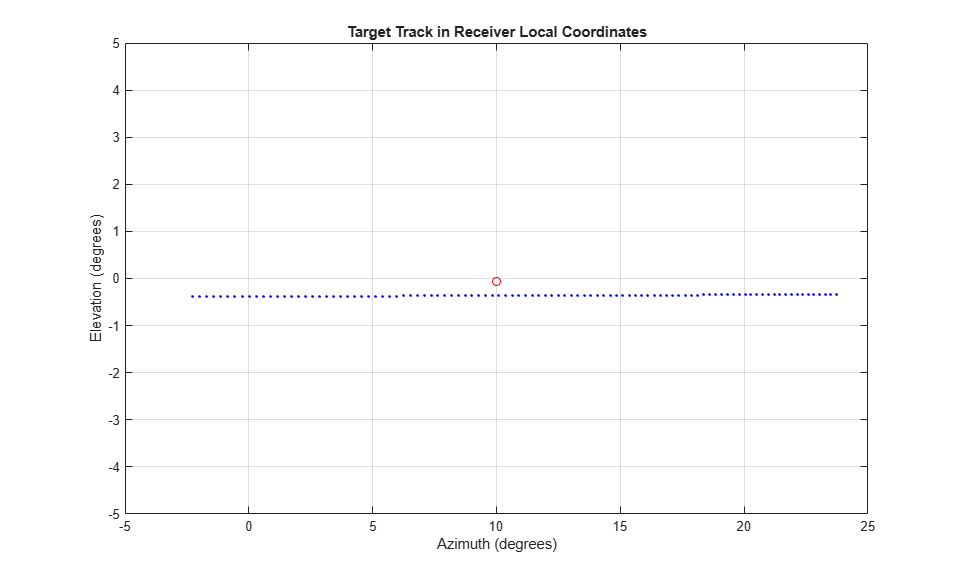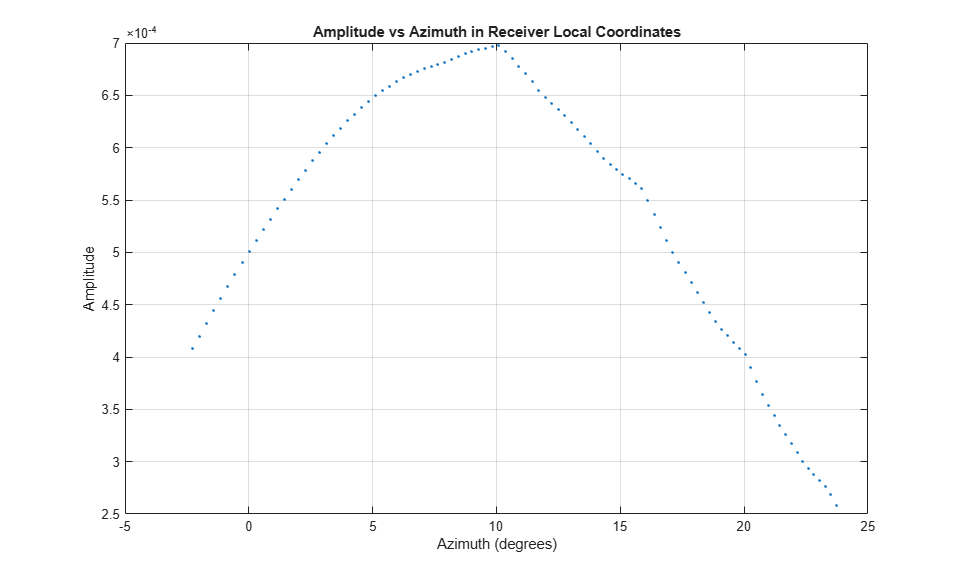# Global and Local Coordinate Systems Radar Example

This example shows how several different coordinate systems come into play when modeling a typical radar scenario. The scenario considered here is a bistatic radar system consisting of a transmitting radar array, a target, and a receiving radar array. The transmitting radar antenna emits radar signals that propagate to the target, reflect off the target, and then propagate to the receiving radar.

Choose a signal frequency of 1 GHz.

```fc = 1e9; c = physconst('LightSpeed'); lam = c/fc; ```

### Create All Radar System Components

First, set up the transmitting radar array. The transmitting array is a 5-by-5 uniform rectangular array (URA) composed of isotropic antenna elements. The array is stationary and is located at the position (50,50,50) meters in the global coordinate system. Although you position arrays in the global system, array element positions are always defined in the array local coordinate system. The transmitted signal strength in any direction is a function of the transmitting angle in the local array coordinate system. Specify the orientation of the array. Without any orientation, local array axes are aligned with the global coordinate system. Choose an array orientation so that the array normal vector points approximately in the direction of the target. Do this by rotating the array 90° around the z-axis. Then, rotate the array slightly by 2° around the y-axis and 1° around the z-axis again.

```antenna = phased.IsotropicAntennaElement('BackBaffled',false); txarray = phased.URA('Element',antenna','Size',[5,5],'ElementSpacing',0.4*lam*[1,1]); txradarAx = rotz(1)*roty(2)*rotz(90); txplatform = phased.Platform('InitialPosition',[50;50;50],... 'Velocity',[0;0;0],'InitialOrientationAxes',txradarAx,... 'OrientationAxesOutputPort',true); radiator = phased.Radiator('Sensor',txarray,'PropagationSpeed',c,... 'WeightsInputPort',true,'OperatingFrequency',fc); steervec = phased.SteeringVector('SensorArray',txarray,'PropagationSpeed',c,... 'IncludeElementResponse',true); ```

Next, position a target approximately 5 km from the transmitter along the global coordinate system y-axis and moving in the x-direction. Typically, you specify radar cross-section values as functions of the incident and reflected ray angles with respect to the local target axes. Choose any target orientation with respect to the global coordinate system.

Simulate a non-fluctuating target, but allow the RCS to change at each call to the `target` method. Set up a simple inline function, `rcsval`, to compute fictitious but reasonable values for RCS at different ray angles.

```tgtAx = rotz(10)*roty(15)*rotx(20); tgtplatform = phased.Platform('InitialPosition',[100; 10000; 100],... 'MotionModel','Acceleration','InitialVelocity',[-50;0;0],'Acceleration',[.015;.015;0],... 'InitialOrientationAxes',tgtAx,'OrientationAxesOutputPort',true); target = phased.RadarTarget('OperatingFrequency',fc,... 'Model','Nonfluctuating','MeanRCSSource','Input port'); rcsval = @(az1,el1,az2,el2) 2*abs(cosd((az1+az2)/2 - 90)*cosd((el1+el2)/2)); ```

Finally, set up the receiving radar array. The receiving array is also a 5-by-5 URA composed of isotropic antenna elements. The array is stationary and is located 150 meters in the z-direction from the transmitting array. The received signal strength in any direction is a function of the incident angle of the signal in the local array coordinate system. Specify an orientation of the array. Choose an orientation so that this array also points approximately in the y-direction towards the target but not quite aligned with the first array. Do this by rotating the array 92° around the z-axis and then 5° around the x-axis.

```rxradarAx = rotx(5)*rotz(92); rxradarAx = rotx(-.2)*rotz(92); rxplatform = phased.Platform('InitialPosition',[50;50;200],... 'Velocity',[0;0;0],'InitialOrientationAxes',rxradarAx,... 'OrientationAxesOutputPort',true); rxarray = phased.URA('Element',antenna','Size',[5,5],'ElementSpacing',0.4*lam*[1,1]); ```

In summary, four different coordinate systems are needed to describe the radar scenario. These are

1. The global coordinate system.

2. A local radar coordinate system defined by the transmitting radar axes.

3. A local coordinate system defined by the target axes.

4. A second local radar coordinate system defined by the receiving radar axes.

The figure here illustrates the four coordinate systems. It is not to scale and does not accurately represent the scenario in the example code.### Specify Transmitted Waveform and Transmitter Amplification

Use a linear FM waveform as the transmitted signal. Assume a sampling frequency of 1 MHz, a pulse repetition frequency of 5 kHz, and a pulse length of 100 microseconds. Set the transmitter peak output power to 1000 W and the gain to 40.0.

```tau = 100e-6; prf = 5000; fs = 1e6; waveform = phased.LinearFMWaveform('PulseWidth',tau,... 'OutputFormat','Pulses','NumPulses',1,'PRF',prf,'SampleRate',fs); transmitter = phased.Transmitter('PeakPower',1000.0,'Gain',40); ```

Create a matched filter from the transmitted waveform.

```filter = phased.MatchedFilter('Coefficients',getMatchedFilter(waveform)); ```

### Specify Propagation Channels

Use free-space models for the propagation of the signal from the transmitting radar to the target and back to the receiving radar.

```channel1 = phased.FreeSpace('OperatingFrequency',fc,... 'TwoWayPropagation',false); channel2 = phased.FreeSpace('OperatingFrequency',fc,... 'TwoWayPropagation',false); ```

### Specify Phaseshift Beamformer

Create a phase-shift beamformer. Point the mainlobe of the beamformer in a specific direction with respect to the local receiver coordinate system. This direction is chosen to be one through which the target passes at some time in its motion. This choice lets us demonstrate how the beamformer response changes as the target passes through the mainlobe.

```rxangsteer = [22.2244;-5.0615]; rxangsteer = [10;-.07]; beamformer = phased.PhaseShiftBeamformer('SensorArray',rxarray,... 'DirectionSource','Property','Direction',rxangsteer,... 'PropagationSpeed',c,'OperatingFrequency',fc); ```

### Simulation loop

Each iteration of the processing loop performs these operations:

2. Generates the LFM waveform.

3. Amplifies the waveform.

4. Radiates the signal from the transmitting antenna array.

5. Propagates the signal to the target.

6. Reflects the signal from the target.

7. Propagates the signal from the target to the receiving antenna array.

8. Collects the received signal at the receiving antenna.

9. Beamforms the arriving signal at the receiving antenna.

10. Match-filters the beamformed signal and finds its peak value.

Transmit 100 pulses of the waveform. Transmit one pulse every 100 milliseconds.

```t = 0; Npulse = 100; dt = 1; ```

Create storage for later plotting.

```azes1 = zeros(Npulse,1); elevs1 = zeros(Npulse,1); azes2 = zeros(Npulse,1); elevs2 = zeros(Npulse,1); rxsig = zeros(Npulse,1); ```

Enter the simulation loop and generate the transmitted waveform.

```for k = 1:Npulse ```
``` t = t + dt; wav = waveform(); ```

Update the positions of the radars and targets. All positions and velocities are defined with respect to the global coordinate system. Because the `OrientationAxesOutputPort` property of the target System object™ is set to `true`, you can obtain the instantaneous local target axes, `tgtAx1`, from the `target` method. These axes are needed to compute the target RCS. The array local axes are fixed so you do not need to update them.

``` [txradarPos,txradarVel] = txplatform(dt); [rxradarPos,rxradarVel] = rxplatform(dt); [tgtPos,tgtVel,tgtAx1] = tgtplatform(dt); ```

Compute the instantaneous range and direction of the target from the transmitting radar. The strength of the transmitted wave depends upon the array gain pattern. This pattern is a function of direction angles with respect to the local radar axes. You can compute the direction of the target with respect to the transmitter local axes using the `rangeangle` function with an optional argument that specifies the local radar axes, `txradarAx`. (Without this additional argument, `rangeangle` returns the azimuth and elevation angles with respect to the global coordinate system).

``` [~,tgtang_tlcs] = rangeangle(tgtPos,txradarPos,txradarAx); ```

An alternative way to compute the direction angles is to first compute them in the global coordinate system and then convert them using the `global2localcoord` function.

Create the transmitted waveform. The transmitted waveform is an amplified version of the generated waveform.

``` txwaveform = transmitter(wav); ```

Radiate the signal in the instantaneous target direction. Recall that the radiator is not steered in this direction but in an angle defined by the steering vector, `txangsteer`. The steering angle is chosen because the target passes through this direction during its motion. A plot will let us see the improvement in the response as the target moves into the main lobe of the radar.

``` txangsteer = [23.1203;-0.5357]; txangsteer = [10;-.07]; sv1 = steervec(fc,txangsteer); wavrad = radiator(txwaveform,tgtang_tlcs,conj(sv1)); ```

Propagate the signal from the transmitting radar to the target. Propagation coordinates are in the global coordinate system.

``` wavprop1 = channel1(wavrad,txradarPos,tgtPos,txradarVel,tgtVel); ```

Reflect the waveform from target back to the receiving radar array. Use the simple angle-dependent RCS model defined previously. Inputs to the rcs-model are azimuth and elevation of the incoming and reflected rays with respect to the local target coordinate system.

``` [~,txang_tgtlcs] = rangeangle(txradarPos,tgtPos,tgtAx1); [~,rxang_tgtlcs] = rangeangle(rxradarPos,tgtPos,tgtAx1); rcs = rcsval(txang_tgtlcs(1),txang_tgtlcs(2),rxang_tgtlcs(1),rxang_tgtlcs(2)); wavreflect = target(wavprop1,rcs); ns = size(wavreflect,1); tm = [0:ns-1]/fs*1e6; ```

Propagate the signal from the target to the receiving radar. As before, all coordinates for signal propagation are expressed in the global coordinate system.

``` wavprop2 = channel2(wavreflect,tgtPos,rxradarPos,tgtVel,rxradarVel); ```

Compute the response of the receiving antenna array in the direction from which the radiation is coming. First, use the `rangeangle` function to compute the direction of the target with respect to the receiving array local axes, by specifying the receiver local coordinate system, `rxradarAx`.

``` [tgtrange_rlcs,tgtang_rlcs] = rangeangle(tgtPos,rxradarPos,rxradarAx); ```

Store the ranges and direction angles for later plotting.

``` azes1(k) = tgtang_tlcs(1); elevs1(k) = tgtang_tlcs(2); azes2(k) = tgtang_rlcs(1); elevs2(k) = tgtang_rlcs(2); ```

Simulate an incoming plane wave at each element from the current direction of the target calculated in the receiver local coordinate system.

``` wavcoll = collectPlaneWave(rxarray,wavprop2,tgtang_rlcs,fc); ```

Beamform the arriving wave. In this scenario, the receiver beamformer points in the direction, `rxangsteer`, specified by the `Direction` property of the `phased.PhaseShiftBeamformer` System object. When the target actually lies in that direction, the response of the array maximized.

``` wavbf = beamformer(wavcoll); ```

Perform match filtering of the beamformed received wave and then find and store the maximum value of each pulse for display. This value will be plotted after the simulation loop ends.

``` y = filter(wavbf); rxsig(k) = max(abs(y)); ```
```end ```

Plot the target track in azimuth and elevation with respect to the transmitter local coordinates. The red circle denotes the direction toward which the transmitter array points.

```plot(azes1,elevs1,'.b') grid xlabel('Azimuth (degrees)') ylabel('Elevation (degrees)') title('Target Track in Transmitter Local Coordinates') hold on plot(txangsteer(1),txangsteer(2),'or') hold off ```Plot the target track in azimuth and elevation with respect to the receiver local coordinates. The red circle denotes the direction toward which the beamformer points.

```plot(azes2,elevs2,'.b') axis([-5.0,25.0,-5.0,5]) grid xlabel('Azimuth (degrees)') ylabel('Elevation (degrees)') title('Target Track in Receiver Local Coordinates') hold on plot(rxangsteer(1),rxangsteer(2),'or') hold off ```Plot the returned signal amplitude vs azimuth in the receiver local coordinates. The value of the amplitude depends on several factors.

```plot(azes2,rxsig,'.') grid xlabel('Azimuth (degrees)') ylabel('Amplitude') title('Amplitude vs Azimuth in Receiver Local Coordinates') ```